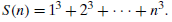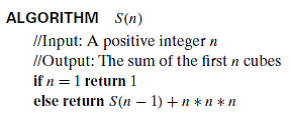Problem

# Consider the following recursive algorithm for computing the sum of the first n cubes:...

Consider the following recursive algorithm for computing the sum of the first n cubes:a. Set up and solve a recurrence relation for the number of times the algorithm’s basic operation is executed.

b. How does this algorithm compare with the straightforward nonrecursive algorithm for computing this sum?

#### Step-by-Step Solution

Solution 1

The recursive algorithm for computing sum of the first $$\mathrm{n}$$ cubes is provided as follows:

ALGORITHM S(n)

// Input: A positive integer $$\mathrm{n}$$

// Output: The sum of the first $$n$$ cubes

if $$n=1$$ return 1

else return $$\mathrm{S}(\mathrm{n}-1)+\mathrm{n}^{*} \mathrm{n}^{*} \mathrm{n}$$

a.

Assume that $$\mathrm{M}(\mathrm{n})$$ be the number of multiplications made by the algorithm. The recurrence relation for $$M(n)$$ is provided as follows:

$$\mathrm{M}(\mathrm{n})=\mathrm{M}(\mathrm{n}-1)+2$$

Solving this recurrence relation by using backward substitutions with $$\mathrm{M}(1)=0$$ is provided as follows:

\begin{aligned} &\mathrm{M}(\mathrm{n})=\mathrm{M}(\mathrm{n}-1)+2 \\ &=[\mathrm{M}(\mathrm{n}-2)+2]+2=\mathrm{M}(\mathrm{n}-2)+2+2 \\ &=[\mathrm{M}(\mathrm{n}-3)+2]+2+2=\mathrm{M}(\mathrm{n}-3)+2+2+2 \\ &=[\mathrm{M}(\mathrm{n}-4)+2]+2+2+2=\mathrm{M}(\mathrm{n}-4)+2+2+2+2 \\ &=\ldots \ldots \text { and so on } \\ &=\mathrm{M}(\mathrm{n}-\mathrm{i})+2 \mathrm{i} \\ &=\ldots \ldots \text { and so on } \\ &=\mathrm{M}(1)+2(\mathrm{n}-1) \\ &=2(n-1) \end{aligned}

b.

The non-recursive algorithm for computing sum of the first $$n$$ cubes is provided as follows: ALGORITHM Non-recursive S(n)

// Computes the sum of the first n cubes non-recursively

// Input: A positive integer $$n$$

// Output: the sum of the first $$n$$ cubes

$$S \leftarrow 1$$

for $$i \leftarrow 2$$ to $$n$$ do

$$\mathrm{S} \leftarrow \mathrm{S}+\mathrm{i}^{*} \mathrm{i}^{*} \mathrm{i}$$

return S

The number of multiplications made by this algorithm $$=\sum_{\mathrm{i}=2}^{\mathrm{n}} 2$$

\begin{aligned} &=2 \sum_{\mathrm{i}-2}^{\mathrm{n}} 1 \\ &=2(\mathrm{n}-1) \end{aligned}

This is the same number as in the recursive version. Only difference is the non-recursive version doesn't carry the time and space overhead associated with recursion's stack.